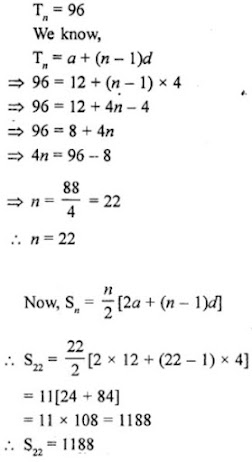# RD Sharma Solutions for Class 10 Maths Chapter 9 Arithmetic Progression Exercise 9.6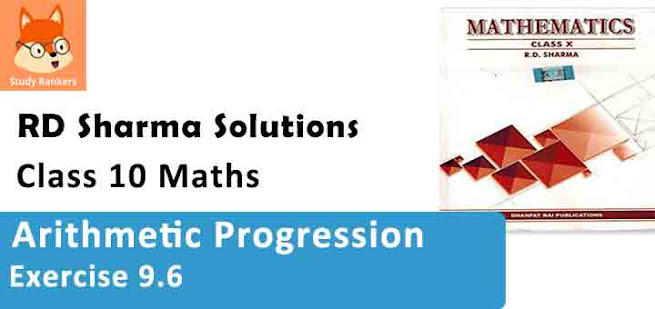1. Find the sum of the following arithmetic progressions:

(i) 50, 46, 42, ...... to 10 terms
(ii) 1, 3, 5, 7, ..... to 12 terms
(iii) 3, 9/2, 6, 15/2, ..... to 25 terms
(iv) 41, 36, 31, ..... to 12 terms
(v) (a + b), (a - b), (a - 3b), ..... to 22 terms
(vi ) (x - y)2 ,(x2 + y2 ), (x + y)2 ,......., to n terms
(vii) (x - y)/(x + y), (3x - 2y)/(x + y), (5x - 3y)/(x + y), .... to n terms
(viii) - 26, -24, -22, ..... to 36 terms

Solution

(i) A.P. is 50, 46, 42,.... to 10 terms.
Here, a = 50, d = 46 - 50 = -4 and n = 10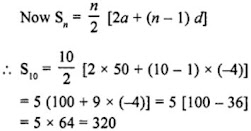(ii) A.P. is 1, 3, 5, 7,.... to 12 terms.
Here, a = 1, d = 3 - 1 = 2 and n = 12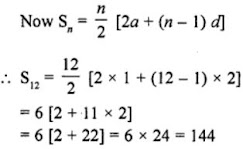(iii) A.P. is 3, 9/2, 6, 15/2,.... to 25 terms.
Here, a = 3, d = 9/2 - 3 = 3/2 and n = 25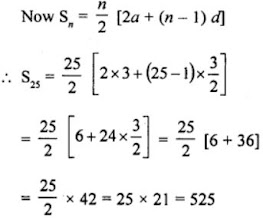(iv) A.P. is 41, 36, 31,.... to 12 terms.
Here, a = 41, d = 36 - 41 = -5 and n = 12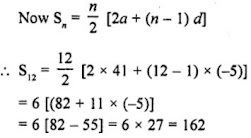(v) A.P. is a+b, a-b, a-3b,.... to 22 terms.
Here, a = a+b, d = a-b - a-b = -2b and n = 22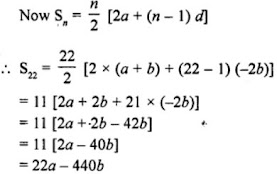(vi) A.P. is (x - y)2, (x2 + y2), (x + y)2 ,....., to n terms.
Here, a = (x - y)2,
d = (x2 + y2) - (x - y)2 = x+ y2 - x2 - y2 + 2xy
n = n terms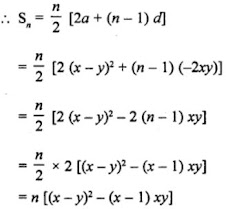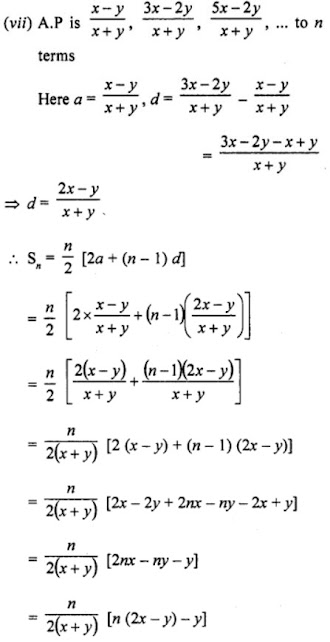(viii) A.P. is -26, -24, -22,.... to 36 terms.
Here, a = -26, d = -24 + 26 = 2 and n = 36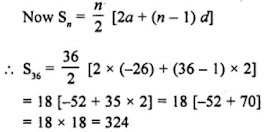2. Find the sum to n term of the A.P. 5, 2, - 1, -4, -7,......

Solution

Given AP is 5, 2, -1, -4, -7,......
Here, a = 5, d = 2 - 5 = -3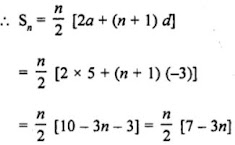3. Find the sum of n terms of an A.P. whose nth terms is given by an = 5 – 6n.
Solution
nth term of the given A.P. is
an = 5 - 6n
First term = a1 = 5 -6×1 = 5 - 6 = -1
a2 = 5 - 6×2 = 5 - 12 = -7
d = a2 - a1 = -7 - (-1) = -7 + 1 = -6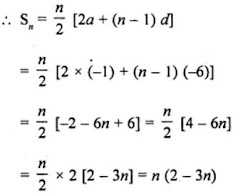4. Find the sum of last ten terms of the A.P.: 8, 10, 12, 14,…, 126.
Solution
For finding the sum of last ten terms, we write the given A.P. in reverse order i.e. 126, 124, 122,...., 12, 10, 8
Here, first term (a) = 126
Common difference (d) = 124 - 126 = -2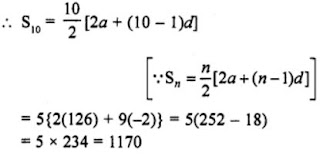5. Find the sum of the first 15 terms of each of the following sequences having nth term as
(i) an = 3 + 4n
(ii) bn = 5 + 2n
(iii) xn = 6 – n
(iv) yn = 9 – 5n
Solution

(i) an = 3+4n, number of terms = 15
a1 = 3 + 4×1 = 3 + 4 = 7 or a = 7
a2 = 3 + 4×2 = 3 + 8 = 11
d = a2 - a1 = 11 - 7 = 4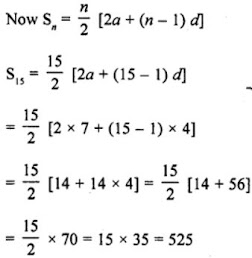(ii) bn = 5 + 2n and number of terms = 15
b1 = 5 + 2×1 = 5 + 2 = 7
b2 = 5 + 2×2 = 5 + 4 = 9
First term (a) = 7 and d = 9 - 7 = 2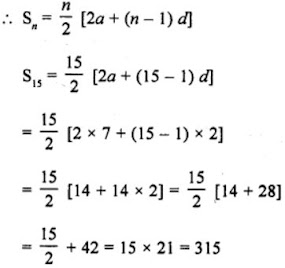(iii) xn = 6 - n and number of terms = 15
x1 = 6 - 1 = 5
x2 = 6 - 2 = 4
First term (a) = 5 and d = 4 - 5 = -1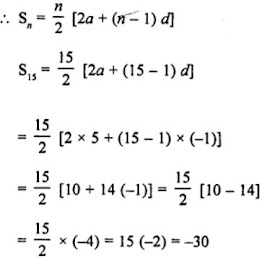(iv) yn = 9 - 5n and number of terms = 15
y1 = 9 - 5×1 = 9 - 5 = 4
y2 = 9 - 5×2 = 9 - 10 = -1
First (a) = 4 and d = y2 - y1 = -1 - 4 = -5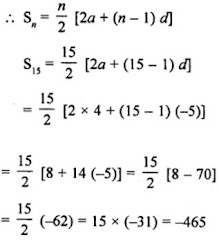6. Find the sum of first 20 terms of the sequence whose nth term is an = An + B.

Solution

nth term = an = An + B and number of terms = 20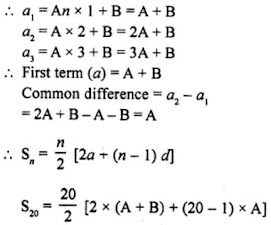= 10[2A + 2B + 19A]
= 10[21A + 2B
= 210A + 20B

7. Find the sum of the first 25 terms of an A.P. whose nth term is given by an = 2 – 3n.

Solution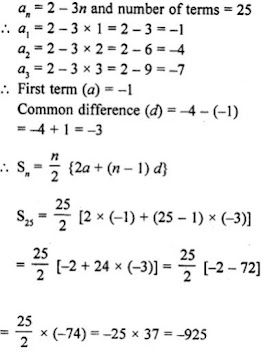8. Find the sum of the first 25 terms of an A.P. whose nth term is given by an = 7 – 3n.

Solution

nth term of an A.P. is an = 7 – 3n and number of terms = 25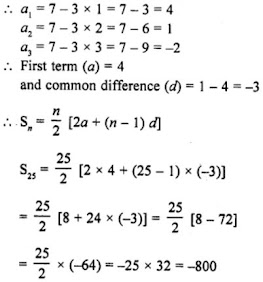9. If the sum of a certain number of terms starting from first term of an A.P. is 25, 22, 19, …, is 116. Find the last term.

Solution

A.P. is given 25, 22, 19,....
Sum of the A.P. = 116
Here, a =25 and d = 22 - 25 = 3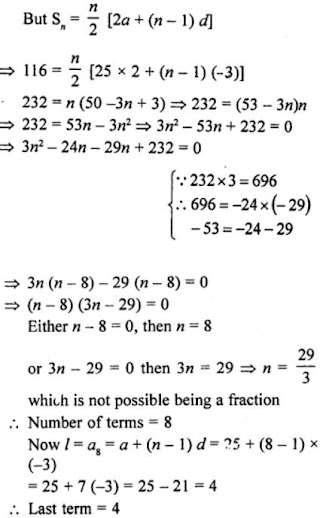10. (i) How many terms of the sequence 18, 16, 14, … should be taken so that their sum is zero ?
(ii) How many terms are there in the A.P. whose first and fifth terms are -14 and 2 respectively and the sum of the terms is 40?
(iii) How many terms of the A.P. 9, 17, 25,… must be taken so that their sum is 636 ?
(iv) How many terms of the A.P. 63, 60, 57, ……… must be taken so that their sum is 693 ?
(v) How many terms of the A.P. 27, 24, 21, …, should be taken so that their sum is zero?

Solution

(i) In the sequence 18, 16, 14,....
Sum = 0
Here, first term (a) = 18 and common difference (d) = 16 - 18 = -2
Let n be the number of terms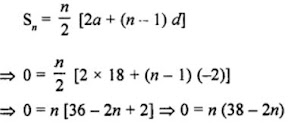Either x = 0, which is not possible.
or, 38 - 2n = 0, then 2n = 38
⇒ n = 19
Number of terms in the sequence = 19.

(ii) First term of an A.P. = -14
and fifth term =2
Sum of terms = 40
Let n be the number of terms, then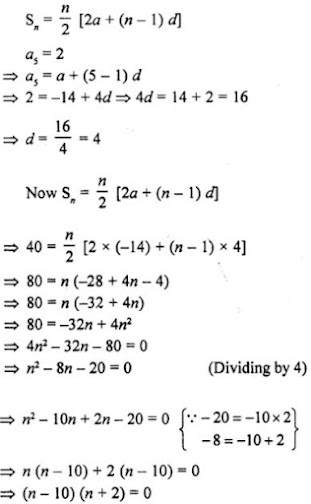Either n - 10 = 0, then n = 10
or, n + 2 = 0
⇒ n = -2 but it is not possible being negative.
Number of terms = 10

(iii) A.P. is 9, 17, 25,....
Let number of terms of the A.P. = n
First term (a) = 9
Common difference (d) = 17 - 9 = 8 and sum of n terms = 636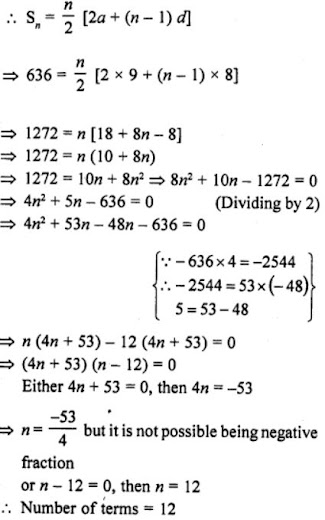(iv) Given A.P. = 63, 60, 57,....
Sum = 693
Let number of terms = n
First term (a) = 63
Common difference (d) = 60 - 63 = -3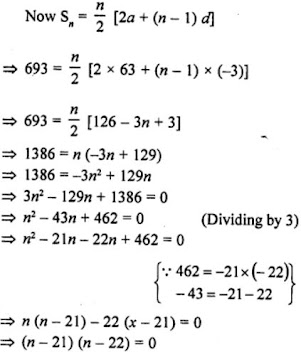Either n - 21 = 0, then n = 21
or, n - 22 = 0, then n = 22
Number of terms = 21 or 22.

(v) A.P. = 27, 24, 21,....
a = 27
d = 24 - 27 = -3
Sum = 0
Let n terms be there in A.P.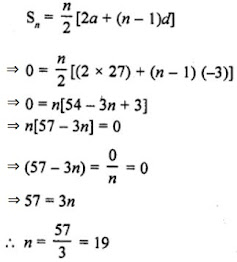11. Find the sum of the first
(i) 11 terms of the A.P. : 2, 6, 10, 14,…
(ii) 13 terms of the A.P. : -6, 0, 6, 12,…
(iii) 51 terms of the A.P.: whose second term is 2 and fourth term is 8.

Solution

(i) A.P. is 2, 6, 10, 14,.... in which first term(a) = 2 and common difference(d) = 6 - 2 = 4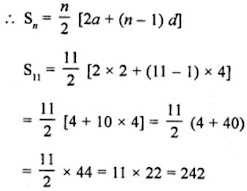(ii) A.P. is 2, 6, 10, 14,.... in which first term(a) = -6 and common difference(d) = 0 - (-6) = 6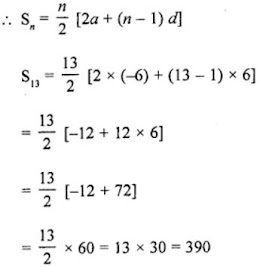(iii) Second term of an A.P. = 2 and fourth term = 8
Let a be the first term and de be the common difference.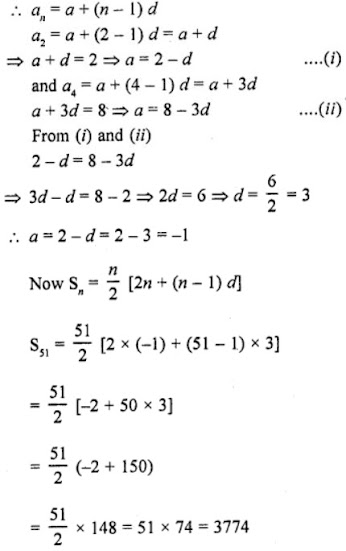12. Find the sum of
(i) the first 15 multiples of 8
(ii) the first 40 positive integers divisible by
(a) 3, (b) 5, (c) 6
(iii) all 3-digit natural numbers which are divisible by 13.
(iv) all 3-digit natural numbers, which are multiples of 11.
(v) all 2-digit natural numbers divisible by 4.

Solution

(i) First 15 multiples of 8 are 8, 16, 24, 32, ..., 120 in which
first term (a) = 8
common difference (d) = 8
and last term = 120(ii) (a) First 40 positive integers divisible by 3 are 3, 6, 9, 12, 15, ..., 120 in which
a = 3,
d = 3
and l = 120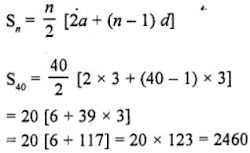(b) 40 multiple of 5 are 5, 10, 15, 25, ... 200 in which
first term (a) = 5
common difference (d) = 5
and last term (l) = 200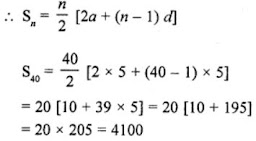(c) 40 multiples of 6 are 6, 12, 18, 24, ... 240 in which
first term (a) = 6
common difference (d) = 6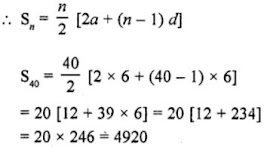(iii) 3-digit numbers which are divisible by 13 will be 104, 117, 130, 143, ... 988
Here, first term (a) = 104 Common difference (d) = 13 and last term (1) = 988
Let n be the numbers of terms.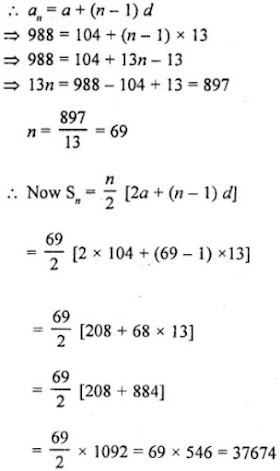(iv) 3-digit numbers which are multiple of 11 are 110, 121, 132, 143, 154, 165, 176, 187, 198, ..., 990
S = 110 + 121 + 132 + 143 +...+ 990
= 11[10+11+12 +13 +...+ 90]
Here, a = 10, d = 1, l = 90
Last term = a + (n - 1) d
90 = 10 + (n - 1)×1
⇒ 90 = 10 + n - 1
⇒ n = 90 - 10 + 1 = 81
⇒ n = 81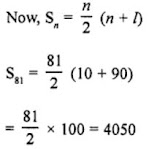(v) The two digits numbers which are divisible by 4 are 12, 16, 20, ..., 96 are in A.P.
Here, a = 12 and d = 4